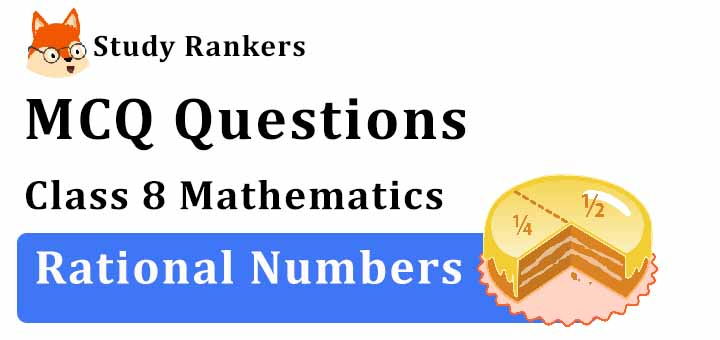## MCQ Questions for Class 8 Maths: Ch 1 Rational Numbers1. The value of ½ x ⅗ is equal to:
(a) ½
(b) 3/10
(c) ⅗
(d) ⅖
► b) 3/10

2. Find the reciprocal of -2.
(a) 2
(b) -2
(c) -1/2
(d) None of these
► c) -1/2

3. How is -28/84 expressed as a rational number with numerator 4?
(a) 4/7
(b) -4/12
(c) 4/12
(d) 4/-7
► (b) -4/12

4. Which of the following statements is true?
(a) Every fraction is a rational number.
(b) Every rational number is a fraction.
(c) Every integer is a rational number.
(d) Both (a) and (c).
► (d) Both (a) and (c).

5. Which of the following is the reciprocal of the reciprocal of a rational number?
(a) -1
(b) 1
(c) 0
(d) The number itself
► (d) The number itself

6. What is the sum of the additive inverse and multiplicative inverse of 2?
(a) 3/2
(b) -3/2
(c) 1/2
(d) -1/2
► (b) -3/2

7. Which among the following is a rational number equivalent to -5/-3 ?
(a) -25/15
(b) 25/-15
(c) 25/15
(d) -25/30
► (c) 25/15

8. Which of the following is the reciprocal of a rational number?
(a) -1
(b) 1
(c) 2
(d) Both a and b
► (d) Both a and b

9. Write the additive inverse of 4/5.
(a) 1
(b) -4/5
(c) 4/5
(d) 0
► (b) -4/5

10. Find the multiplicative inverse of 1/4.
(a) 4
(b) -1/4
(c) -4
(d) 1/4
► (a) 4

11. What is the additive inverse of -2/3?
(a) 0
(b) 1
(c) 2/3
(d) -2/3
► (c) 2/3

12. The value of ½ + ¼ is equal to:
(a) ¾
(b) 3/2
(c) ⅔
(d) 1
► (a) ¾

13. Which of the following is the identity element under addition?
(a) 1
(b) -1
(c) 0
(d) None of these
► (c) 0

14. A number which can be written in the form, p/q where p and q are integers and _____ is called a rational number.
(a) q = 0
(b) q ≠ 0
(c) q = 1
(d) none of these
► (b) q ≠ 0

15. Which of the following statements is true?
(a) Every fraction is a rational number.
(b) Every rational number is a fraction.
(c) Every integer is a rational number.
(d) Both (a) and (c).
► (d) Both (a) and (c).

16. Which of the following is the Multiplicative identity for rational numbers?
(a) 1
(b) -1
(c) 0
(d) None of these
► (a) 1

17. Find the multiplicative inverse of -13.
(a) 13
(b) -13
(c) -1/13
(d) 12
► (c) -1/13

18. ________ is not associative for rational numbers.
(a) Subtraction or Division
(d) Multiplication or Division
► (a) Subtraction or Division

19. Which number is in the middle if -1/6, 4/9, 6/-7, 2/5 and -3/4 arranged in descending order?
(a) 2/5
(b) 4/9
(c) -1/6
(d) -6/7
► (c) -1/6

20. Which of the following is the Multiplicative identity for rational numbers?
(a) 1
(b) -1
(c) 0
(d) None of these
► (a) 1

21. Which of the following forms a pair of equivalent rational numbers?
(a) 24/40 and 35/50
(b) -25/35 and 55/-77
(c) -8/15 and -24/48
(d) 9/72 and -3/21
► (b) -25/35 and 55/-77

22. What should be subtracted from -7/11 to get −2?
(a) 15/11
(b) -15/11
(c) 29/11
(d) -29/11
► (a) 15/11